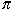Name: Douglas Who is asking: Other Level: All Question: If you know how far around a circle is (say earth) 25000 miles how do you calculate the distance across? Thanks Doug Hi Douglas, This follow from the wonderful property of circles. No matter how large or small a circle you have, if you divide the distance around (the circumference) by the distance across (the diameter) the answer is always p. That is  circumference/diameter =The numberis approximately 3.1416 and hence if the circumference is 25000 miles as in your example then  25000/diameter =and the diameter is then  25000/which is approximately 7958 miles. Cheers, Penny Go to Math Central